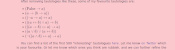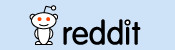mscroggs.co.uk
mscroggs.co.uksubscribe

# Blog

2016-10-08
During my Electromagnetic Field talk this year, I spoke about @mathslogicbot, my Twitter bot that is working its way through the tautologies in propositional calculus. My talk included my conjecture that the number of tautologies of length $$n$$ is an increasing sequence (except when $$n=8$$). After my talk, Henry Segerman suggested that I also look at the number of contradictions of length $$n$$ to look for insights.
A contradiction is the opposite of a tautology: it is a formula that is False for every assignment of truth values to the variables. For example, here are a few contradictions:
$$\neg(a\leftrightarrow a)$$ $$\neg(a\rightarrow a)$$ $$(\neg a\wedge a)$$ $$(\neg a\leftrightarrow a)$$
The first eleven terms of the sequence whose $$n$$th term is the number of contradictions of length $$n$$ are:
$$0, 0, 0, 0, 0, 6, 2, 20, 6, 127, 154$$
This sequence is A277275 on OEIS. A list of contractions can be found here.
For the same reasons as the sequence of tautologies, I would expect this sequence to be increasing. Surprisingly, it is not increasing for small values of $$n$$, but I again conjecture that it is increasing after a certain point.

### Properties of the sequences

There are some properties of the two sequences that we can show. Let $$a(n)$$ be the number of tautolgies of length $$n$$ and let $$b(n)$$ be the number of contradictions of length $$n$$.
First, the number of tautologies and contradictions, $$a(n)+b(n)$$, (A277276) is an increasing sequence. This is due to the facts that $$a(n+1)\geq b(n)$$ and $$b(n+1)\geq a(n)$$, as every tautology of length $$n$$ becomes a contraction of length $$n+1$$ by appending a $$\neg$$ to be start and vice versa.
This implies that for each $$n$$, at most one of $$a$$ and $$b$$ can be decreasing at $$n$$, as if both were decreasing, then $$a+b$$ would be decreasing. Sadly, this doesn't seem to give us a way to prove the conjectures, but it is a small amount of progress towards them.

### Similar postsLogic bot, pt. 2Logic botInteresting tautologiesHow OEISbot works

Comments in green were written by me. Comments in blue were not written by me.